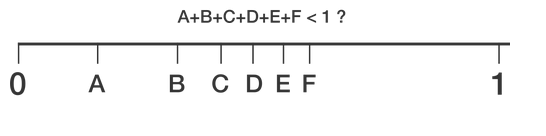# Six random variables

Probability Level 3Six variables $x_i$ are chosen uniformly at random in the interval $[0,1]$.

Find the probability that $x_1 + x_2 + x_3 + x_4 + x_5 + x_6 < 1.$ If the answer is of the form $\frac{a}{b}$, where $a$ and $b$ are coprime positive integers, what is $a+b?$

###### Inspired by Brian Charlesworth
×

Problem Loading...

Note Loading...

Set Loading...